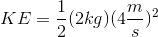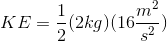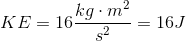# GED Science : Kinetic Energy

## Example Questions

### Example Question #5 : Physics

A ball that has a mass of 2kg is launched upward at a velocity of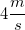. What is the initial kinetic energy of the ball?

Assume the ball is traveling in a vacuum.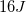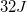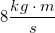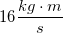Explanation:

Kinetic energy (measured in Joules) can be solved using the equation: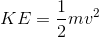We are given both the mass of the projectile (2kg) and its velocity (). Using these values, we can solve the equation.